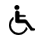Pristupačnost

Poll
Repository
Repository is empty
Algorithms and Programming
 Code: 60214 ECTS: 6.0 Lecturers in charge: prof. dr. sc. Kristian Vlahoviček - Lectures doc. dr. sc. Rosa Karlić - Lectures Lecturers: Dunja Glavaš - Practicum Rosa Karlić - Practicum Maja Kuzman - Practicum Take exam: Studomat English level: 1,0,0 All teaching activities will be held in Croatian. However, foreign students in mixed groups will have the opportunity to attend additional office hours with the lecturer and teaching assistants in English to help master the course materials. Additionally, the lecturer will refer foreign students to the corresponding literature in English, as well as give them the possibility of taking the associated exams in English.

### 1. komponenta

Lecture typeTotal
Lectures 15
Practicum 30
Description:
LEARNING OUTCOMES:
1. Use RStudio integrated development environment for writing program code in the R programming language.
2. Create variables of any R language base types (vector, matrix, factor, list, data frame) and do simple manipulations with data stored in them.
3. Create basic data visualizations (line and bar plots, histograms) by using functions from the R base package.
4. Create simple and advanced (replacement and infix) R functions.
5. Use control structures of R programming language.
6. Write readable program code so that it is easy to maintain and upgrade by third parties.
7. Use external R code packages.
8. Load data from formatted and unformatted sources such as web pages, csv files etc.
9. Use basic commands of the Linux command line interface.
10. Analyze simple algorithms in terms of their time and space complexity.
CLASS SYLLABUS:
Class aims to acquaint biology students with fundamentals of programming and algorithms needed to analyze and solve biological problems by using the R programming language.
CLASSES:
1. short history of R programming language and reasons behind the rise of its usage in biology and data science;
2. overview of basic integrated development environment (IDE) packed with R programming language;
3. getting acquainted with RStudio IDE;
4. writing R scripts;
5. good programming practices (code formatting and writing code comments);
6. variable types in R;
7. writing basic functions in R; return value of the function;
8. control structures in R (if, if else, for, while);
9. vectorized operations in R and their advantages over non vectorized operations;
10. data visualization by using the functions in R base package;
11. using basic Linux command line tools (cd, ls, du, grep, chmod, find);
12. regular expressions; using regular expressions to search through and manipulate text data;
13. using external R packages;
14. packages microbenchmark and dplyr;
15. debugging in RStudio IDE
PRACTICAL PART OF THE COURSE:
In practical part of the course students are expected to apply the knowledge gained in classes to solve six groups of exercises. Each students solution is graded and used in forming part of the final grade for the class. Each student gets individual feedback with comments for each solved exercise. All groups of exercises are solved in RStudio IDE by using the R programming language except for one (21. 25. hour) which is solved by using the Linux operating system console.
1.-5. writing simple scripts; creating sequences; creating simple functions; creating simple line plots; vector variables; recursive functions; writing readable program code
6.-10. using functions for generating random numbers from variety of standard distributions, calculating probability density and cumulative distribution; creating histograms; matrix data type; data frame; list; replacement functions;
11.-15. loading data from csv formatted text file; making changes into and searching through text data by using regular expressions; loading data from unformatted text files; simulating stochastic events;
16.-20. infix functions; advanced manipulations of data frames; using the dplyr package for manipulating the data regarding intron position in the genome; expression data analysis;
21.-25. connecting to Linux console of a remote computer; working with Linux command line; data manipulation by using the Linux command line;
26.-30. Programming complex functions; using the RStudio IDE for debugging purposes; breaking complex problem into smaller parts (functions); execution time comparison by using the microbenchmark package;
Literature:
 3. semester Izborni predmeti - Računalna biologija - Mandatory studij - Molecular Biology
Consultations schedule:
News